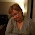Saturday, 26 January 2019

Si5351 Quadrature Clock Output down to 3MHz

Credit to Brian Harper M1CEM and Miguel Bartié PY2OHH

Step 1. Edit si5351.h file. Change the SI5351_PLL_VCO_MIN to 380000000, i.e.,

#define SI5351_PLL_VCO_MIN              380000000

Step 2. Example code snippet:

volatile long freq = 3500000;
volatile int Even_Divisor = 0;
volatile int oldEven_Divisor = 0;

void EvenDivisor()
{
if (freq < 6850000)
{
Even_Divisor = 126;
}
if ((freq >= 6850000) && (freq < 9500000))
{
Even_Divisor = 88;
}
if ((freq >= 9500000) && (freq < 13600000))
{
Even_Divisor = 64;
}
if ((freq >= 13600000) && (freq < 17500000))
{
Even_Divisor = 44;
}
if ((freq >= 17500000) && (freq < 25000000))
{
Even_Divisor = 34;
}
if ((freq >= 25000000) && (freq < 36000000))
{
Even_Divisor = 24;
}
if ((freq >= 36000000) && (freq < 45000000)) {
Even_Divisor = 18;
}
if ((freq >= 45000000) && (freq < 60000000)) {
Even_Divisor = 14;
}
if ((freq >= 60000000) && (freq < 80000000)) {
Even_Divisor = 10;
}
if ((freq >= 80000000) && (freq < 100000000)) {
Even_Divisor = 8;
}
if ((freq >= 100000000) && (freq < 146600000)) {
Even_Divisor = 6;
}
if ((freq >= 150000000) && (freq < 220000000)) {
Even_Divisor = 4;
}
}

void SendFrequency()
{
EvenDivisor();
si5351.set_freq_manual(freq * SI5351_FREQ_MULT, Even_Divisor * freq * SI5351_FREQ_MULT, SI5351_CLK0);
si5351.set_freq_manual(freq * SI5351_FREQ_MULT, Even_Divisor * freq * SI5351_FREQ_MULT, SI5351_CLK2);
si5351.set_phase(SI5351_CLK0, 0);
si5351.set_phase(SI5351_CLK2, Even_Divisor);

if(Even_Divisor != oldEven_Divisor)
{
si5351.pll_reset(SI5351_PLLA);
oldEven_Divisor = Even_Divisor;
}
}

1.You should only do a pll_reset when the even_divisor is changed. A pll_reset will make clicks. Hans Summers wrote a little about it here: https://groups.io/g/BITX20/topic/si5351_quadrature_vfo/22076271?20,0,0,0::recentpostdate%2Fsticky,,,20,2,0,22076271

2.Thanks Niel. That's exactly I found too. I've changed my code to only do the reset on Even_Divisor change. I'll update the code above IDC.

Thanks again.

Charlie

3.Good day !!
We are Christian Organization formed to help people in need of help,such as
financial assistance, Do you need a loan to pay your bills? Do you need
Personal Business Car or Student loans? Need a loan for various other